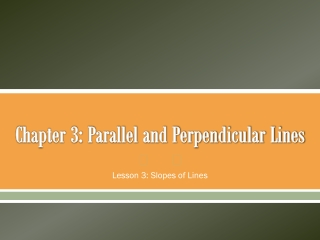DownloadDownload PresentationChapter 3: Parallel and Perpendicular Lines

# Chapter 3: Parallel and Perpendicular Lines

Télécharger la présentation## Chapter 3: Parallel and Perpendicular Lines

- - - - - - - - - - - - - - - - - - - - - - - - - - - E N D - - - - - - - - - - - - - - - - - - - - - - - - - - -
##### Presentation Transcript

1. Chapter 3: Parallel and Perpendicular Lines Lesson 3: Slopes of Lines

2. Slope • The ratio of the vertical rise over the horizontal run • Can be used to describe a rate of change • Two non-vertical lines have the same slope if and only if they are parallel • Two non-vertical lines are perpendicular if and only if the product of their slopes is -1

3. Rise = 0 zero slope (horizontal line) Run = 0 undefined (vertical line) Parallel = same slope Perpendicular = one slope is the reciprocal and opposite sign of the other Example:find the slope of a line containing (4, 6) and (-2, 8) Slope

4. Example Find the slope of the line.

5. Example Find the slope of the line.

6. Example Find the slope of the line.

7. Example • Determine whether FG and HJ are parallel,perpendicular, or neither for F(1, –3), G(–2, –1), H(5, 0), and J(6, 3). (DO NOT GRAPH TO FIGURE THIS OUT!!)

8. Example • Determine whether AB and CD are parallel,perpendicular, or neither for A(–2, –1), B(4, 5), C(6, 1), and D(9, –2)

9. A. Graph the line that contains Q(5, 1) and is parallel to MN with M(–2, 4) and N(2, 1). B. Graph the line that contains (-1, -3) and is perpendicular to MN for M(–3, 4) and N(5, –8)? Examples